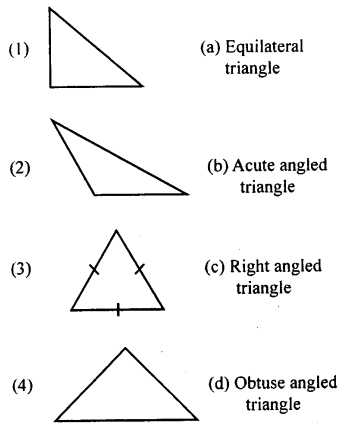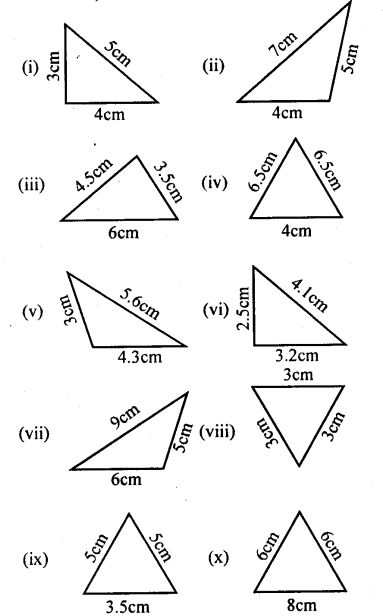# KSEEB Solutions for Class 8 Maths Chapter 6 Theorems on Triangles Ex 6.1

Students can Download Maths Chapter 6 Theorems on Triangles Ex 6.1 Questions and Answers, Notes Pdf, KSEEB Solutions for Class 8 Maths helps you to revise the complete Karnataka State Board Syllabus and score more marks in your examinations.

## Karnataka Board Class 8 Maths Chapter 6 Theorems on Triangles Ex 6.1

Question 1.
Match the following1 – c
2 – d
3 – a
4 – b.

Question 2.
Based on the sides, classify the following triangles (figures not drawn of the scales)